## 1 Introduction

Let X be a smooth irreducible projective variety and let H be a very ample divisor on X. A vector bundle $${{\mathcal {E}}}$$ on X is said to be an Ulrich vector bundle with respect to the polarization H if $$h^i({{\mathcal {E}}}(-pH))=0$$ for all $$i \geqslant 0$$ and all $$1 \leqslant p \leqslant \dim (X)$$.

Ulrich bundles first appeared in commutative algebra in the 1980s, where they have been considered because they enjoy some extremal cohomological properties. After that the attention of the algebraic geometers on these bundles has been carried by the beautiful paper , where, among other things, the authors compute the Chow form of a projective variety X using Ulrich bundles on X, if they exist.

In recent years there has been a good amount of work on Ulrich bundles (for surveys see, for instance, [4, 11, 13]), mainly investigating the following problems: given any polarization H on a variety X, does there exist an Ulrich vector bundle with respect to H? Or, even more generally, given a variety X, does there exist a very ample line bundle H on X and an Ulrich vector bundle on X with respect to H? What is the smallest possible rank for an Ulrich bundle on a given variety X? If Ulrich bundles exist, are they stable, and what are their moduli?

Although a lot is known about these problems for some specific classes of varieties (curves, Segre, Veronese, Grassmann varieties, rational scrolls, complete intersections, some classes of surfaces like Del Pezzo, abelian, K3 surfaces, some surfaces of general type, etc.) the above questions are still open in their full generality even for surfaces. In particular, the question for which pairs (XH) there are Ulrich line bundles on X with respect to H is open, though this occurrence seems to be rather rare. Moreover, in the few known cases in which they have been proved to exist, except the case of curves, they are finitely many and of a low number.

In the present paper we investigate the existence of Ulrich bundles on the blow-up $$X_n$$ of the complex projective plane at n very general points with respect to line bundles $$\xi _{n,m}$$ corresponding to curves of degree m passing simply through the n blown-up points, with $$m\leqslant 2\sqrt{n}$$ and such that the line bundle in question is very ample. Surprisingly enough, our results show that such a surface carries several Ulrich line bundles, and actually their number increases and tends to infinity with n, see Theorem 3.1 and Corollary 3.2. In Theorem 4.2 we classify all these line bundles for $$7\leqslant n\leqslant 10$$ and $$m=4$$ (the cases $$n\leqslant 6$$ and $$m=3$$ corresponding to the Del Pezzo case being well known already, see [12, 19]). Then, making iterated extensions and deforming, we prove the existence of slope-stable rank-r Ulrich vector bundles on $$X_n$$ for $$n\geqslant 2$$ and any $$r \geqslant 1$$ (see Theorem 5.8), we compute the dimension of the moduli spaces of the bundles in question and prove that they are reduced. These computations show that $$X_n$$, with $$n\geqslant 2$$, is Ulrich wild.

Recall that a variety X is said to be Ulrich wild if it possesses families of dimension p of pairwise non-isomorphic, indecomposable, Ulrich bundles for arbitrarily large p (for the introduction of the notion of Ulrich, or more generally, of Cohen–Macaulay wildness, see, for instance, [14, 16]). Note that in the literature there are only very few cases of varieties known to carry stable Ulrich bundles of infinitely many ranks, and even fewer of any rank (namely, curves and Del Pezzo surfaces). Note that it is known that not all varieties can support Ulrich vector bundles of every rank (see e.g. [10, Cor. 2.], [1, Cor. 3.2], [4, §4] and [8, Cor. 3.7 and 3.9]). In [2, Cor. 3.8] the existence of rank 2 Ulrich vector bundles is proved on the blown-up planes with respect to an ample line bundle of the form $$\xi _{n,m}$$, without investigating stability or moduli. The description of Ulrich vector bundles on some surfaces of type $$X_n$$ which are not Del Pezzo surfaces and the related Ulrich wildness can be found in . The fact that $$X_n$$ supports slope stable rank-two Ulrich bundles and is Ulrich wild for each $$n\geqslant 2$$ is a particular case of the results in .

It is an interesting problem to study the presence of Ulrich (line) bundles on blow-ups at very general points of other surfaces than the projective plane. The ideas in this paper might be useful to attack this problem.

Ciro Ciliberto and Flaminio Flamini are members of GNSAGA of the Istituto Nazionale di Alta Matematica “F. Severi” and acknowledge support from the MIUR Excellence Department Project awarded to the Department of Mathematics, University of Rome Tor Vergata, CUP E83C18000100006. Andreas Leopold Knutsen acknowledges support from the Trond Mohn Foundation Project “Pure Mathematics in Norway”, grant 261756 of the Research Council of Norway and the Meltzer Foundation.

## 2 Preliminaries

2.1 In this paper we will denote by $$\pi : X_n\rightarrow {{\mathbb {P}}}^2$$ the blow-up of the complex projective plane at $$n\geqslant 1$$ very general points $$p_1,\ldots , p_n$$. (The n points are supposed to be very general because one has to take off the a priori countably many closed subsets of n-tuples of points for which the very ampleness of the line bundles $$\xi _{m,n}$$ considered below or the property of imposing independent conditions to suitable linear systems are not satisfied.) We will denote by $$E_1,\ldots , E_n$$ the exceptional divisors contracted by $$\pi$$ to $$p_1,\ldots , p_n$$, respectively, and by L the pull-back via $$\pi$$ of a general line of $${{\mathbb {P}}}^2$$. For integers $$d, m_1,\ldots , m_n$$, consider the linear system

\begin{aligned} \left| dL-\sum _{i=1}^nm_iE_i\right| \end{aligned}
(1)

on $$X_n$$. If $$d, m_1,\ldots , m_n$$ are non-negative, this is the strict transform on $$X_n$$ of the linear system of curves of degree d in $${{\mathbb {P}}}^2$$ with multiplicities at least $$m_1,\ldots , m_n$$ at $$p_1,\ldots , p_n$$, respectively. If one of the integers $$m_1,\ldots , m_n$$ is negative; for example, $$m_1$$ is negative, then this means that $$m_1E_1$$ is in the fixed part of the linear system (1), if this system is non-empty. We will denote the linear system (1), or the corresponding line bundle $${{\mathcal {O}}}_{X_n}(dL-\sum _{i=1}^nm_iE_i)$$, by

\begin{aligned} (d;m_1,\ldots , m_n) \end{aligned}

and we will use exponential notation for repeated multiplicities.

In the sequel we will consider various linear systems of the form $$(d;m_1,\ldots , m_n)$$ as above (cf. e.g. Lemma 2.2, Proposition 2.3, Theorems 3.1, 4.2 and Sect. 5). We will consider the linear systems

\begin{aligned} \xi _{n,m}:=(m;1^n), \end{aligned}

i.e. in the notation $$(d;m_1,\ldots , m_n)$$, we have $$d = m$$ and $$m_i = 1$$ for all $$i = 1, \ldots , n$$.

The line bundle $$\xi _{n,m}$$ is ample as soon as $$n\geqslant 3$$ and $$\xi _{n,m}^2=m^2-n>0$$ (see [17, Cor.p. 154]) and it is very ample if $$m\geqslant 5$$ and

\begin{aligned} m\geqslant 2\sqrt{n+4}-3 \end{aligned}

(see [20, Thm. 1]). This result is not optimal and a natural conjecture is that $$\xi _{n,m}$$ is very ample as soon as

\begin{aligned} \frac{m(m+3)}{2}-n\geqslant 5 \end{aligned}

(see again [20, Conj. p. 2521]). Moreover, it is classically known that $$\xi _{10,4}$$ is very ample, the image of $$X_{10}$$ via the map determined by $$\xi _{10,4}$$ is the famous smooth Bordiga surface of degree 6 in $${\mathbb {P}}^4$$. Accordingly $$\xi _{n,4}$$ is very ample if $$n\leqslant 10$$. In any event, if $$m_n$$ is the minimum m such that $$\xi _{n,m}$$ is very ample, we have

\begin{aligned} m_n\leqslant \left\lceil { 2\sqrt{n+4}-3}\right\rceil \end{aligned}
(2)

if $$n\geqslant 3$$, whereas $$m_1=2$$ and $$m_2=3$$.

We will need the following:

### Lemma 2.1

If $$n\geqslant 3$$, there is an $$m< 2\sqrt{n}$$ such that $$\xi _{n,m}$$ is very ample.

### Proof

To prove the assertion one has to prove that $$2\sqrt{n}> m_n$$. By (2), this is implied by $$2\sqrt{n}> \left\lceil { 2\sqrt{n+4}-3}\right\rceil$$, which holds if $$2\sqrt{n}\geqslant 2\sqrt{n+4}-2$$. This is true as soon as $$n\geqslant 3$$. $$\square$$

2.2 Given a linear system $${{\mathcal {L}}}=(d;m_1,\ldots , m_n)$$, its virtual dimension is

\begin{aligned} \dim _v({{\mathcal {L}}})=\frac{d(d+3)}{2}-\sum _{i=1}^n\frac{m_i(m_i+1)}{2}. \end{aligned}

We have $$\dim ({{\mathcal {L}}})\geqslant \dim _v({{\mathcal {L}}})$$, and if equality holds we will say that the system $${{\mathcal {L}}}$$ is regular.

One computes

\begin{aligned} \dim _v({{\mathcal {L}}})=\chi ({{\mathcal {L}}})-1 \end{aligned}

hence, by the Riemann–Roch theorem, one has

\begin{aligned} \dim ({{\mathcal {L}}})=\dim _v({{\mathcal {L}}})+h^1({{\mathcal {L}}})-h^2({{\mathcal {L}}}). \end{aligned}

Since $$h^2({{\mathcal {L}}})=h^0(K_n\otimes {{\mathcal {L}}}^\vee )$$, where $$K_n=(-3;-1^n)$$ denotes the canonical bundle of $$X_n$$, and

\begin{aligned} K_n\otimes {{\mathcal {L}}}^\vee =(-3-d;-m_1-1,\ldots , -m_n-1) \end{aligned}

one has $$h^2({{\mathcal {L}}})=0$$ as soon as $$d>-3$$. In this case one has

\begin{aligned} \dim ({{\mathcal {L}}})=\dim _v({{\mathcal {L}}})+h^1({{\mathcal {L}}}) \end{aligned}
(3)

and $${{\mathcal {L}}}$$ is regular if and only if $$h^1({{\mathcal {L}}})=0$$.

### Lemma 2.2

Let $${{\mathcal {L}}}$$ be a linear system on $$X_n$$ of the form

\begin{aligned} (d; 1^h,0^k, (-1)^{n-h-k}) \end{aligned}

where $$d\geqslant 0$$. If $$\dim _v({{\mathcal {L}}})\geqslant -1$$, then $${{\mathcal {L}}}$$ is regular.

### Proof

Set $$E=E_{h+k+1}+\cdots +E_n$$. For each curve $$E_i\simeq {{\mathbb {P}}}^1$$, for $$h+k+1\leqslant i\leqslant n$$ one has $${{\mathcal {L}}}\cdot E_i=-1$$, hence E is in the fixed part of $${{\mathcal {L}}}$$, if this is non-empty. So we have $$\dim ({{\mathcal {L}}})=\dim ({{\mathcal {L}}}(-E))$$. The linear system $${{\mathcal {L}}}(-E)$$ is $$(d; 1^h,0^k)$$. One has $$\dim _v({{\mathcal {L}}})=\dim _v({{\mathcal {L}}}(-E))$$. By $$\dim _v({{\mathcal {L}}}(-E))\geqslant -1$$ and by the very generality of the imposed simple base points $$p_1,\ldots , p_h$$, it follows that $${{\mathcal {L}}}(-E)$$ is regular. Hence, we have

\begin{aligned} \dim ({{\mathcal {L}}})=\dim ({{\mathcal {L}}}(-E))=\dim _v({{\mathcal {L}}}(-E))=\dim _v({{\mathcal {L}}}) \end{aligned}

as wanted. $$\square$$

2.3 We will use the following result:

### Proposition 2.3

Consider a linear system of the form

\begin{aligned} {{\mathcal {L}}}_{d,n,\delta ,k}=(d;2^\delta ,1^k, 0^{n-\delta -k}) \end{aligned}

(and permuted multiplicities), with $$k\geqslant 1$$. If

\begin{aligned} \dim _v({{\mathcal {L}}}_{d,n,\delta ,k})=\frac{d(d+3)}{2}-3\delta -k\geqslant 0 \end{aligned}

then $${{\mathcal {L}}}_{d,n,\delta ,k}$$ is regular, i.e.

\begin{aligned} h^1(X_n,{{\mathcal {L}}}_{d,n,\delta ,k})=0 \end{aligned}

and the general curve in $${{\mathcal {L}}}_{d,n,\delta ,k}$$ on $$X_n$$ is smooth and irreducible.

### Proof

This is a straightforward application of [9, Thm. 2.3] (see also [3, Cor. 4.6] and [7, Thms. 1.1, 1.3 and 1.4]). $$\square$$

2.4 Let S be a smooth irreducible projective surface and let H be a very ample divisor on S. It is well known and easily seen that $${{\mathcal {O}}}_S$$ is Ulrich with respect to H if and only if $$S={\mathbb {P}}^2$$ and H is a line.

We will need the following proposition (cf. also [6, Prop. (2.1)]):

### Proposition 2.4

Let S be a smooth irreducible projective surface and let H be a very ample divisor on S.

A line bundle $${{\mathcal {L}}}\not \simeq {{\mathcal {O}}}_S$$ is Ulrich if and only if it is of the form $${{\mathcal {L}}}={{\mathcal {O}}}_S(C)$$, where C is an effective divisor on S satisfying

1. (i)

$$C \cdot H = \frac{1}{2} H \cdot (3H+K_S)$$;

2. (ii)

$$\frac{1}{2} (C^2 - C \cdot K_S) + \chi ({{\mathcal {O}}}_S)-H^2=0$$;

3. (iii)

$$h^1({{\mathcal {O}}}_C(K_S+H))=0$$;

4. (iv)

the restriction map $$r: H^0({{\mathcal {O}}}_S(K_S+2H)) \rightarrow H^0({{\mathcal {O}}}_C(K_S+2H))$$ is injective or surjective.

Moreover, the divisor C can be taken to be a smooth curve.

We will say that $${{\mathcal {L}}}$$ as above is determined by C.

### Proof

It is well known that an Ulrich bundle is globally generated, whence it is of the form $${{\mathcal {L}}}={{\mathcal {O}}}_S(C)$$ for C an effective nonzero divisor, which can even be taken to be a smooth curve. Hence, $${{\mathcal {L}}}$$ is Ulrich if and only if $$h^i({{\mathcal {O}}}_S(C-jH))=0$$ for $$i=0,1,2, \; j=1,2$$. From the short exact sequence

\begin{aligned} 0 \longrightarrow {{\mathcal {O}}}_S(-jH) \longrightarrow {{\mathcal {O}}}_S(C-jH) \longrightarrow \omega _C(-K_S-jH) \longrightarrow 0 \end{aligned}
(4)

we see that $${{\mathcal {L}}}$$ is Ulrich if and only if

\begin{aligned}{} & {} h^0(\omega _C(-K_S-jH))=0, \quad j=1,2, \end{aligned}
(5)
\begin{aligned}{} & {} \text{ the } \text{ coboundary } \text{ maps } \; H^1(\omega _C(-K_S-jH)) \rightarrow H^2({{\mathcal {O}}}_S(-jH)) \nonumber \\{} & {} \text{ are } \text{ isomorphisms }, \quad j=1,2. \end{aligned}
(6)

Clearly the vanishing for $$j=2$$ in (5) is implied by the one for $$j=1$$. Thus, by Serre duality (5)–(6) are equivalent to

\begin{aligned}{} & {} h^1({{\mathcal {O}}}_C(K_S+H))=0, \end{aligned}
(7)
\begin{aligned}{} & {} \text{ the } \text{ restriction } \text{ maps } \; r_j: H^0({{\mathcal {O}}}_S(K_S+jH)) \rightarrow H^0({{\mathcal {O}}}_C(K_S+jH)) \nonumber \\{} & {} \text{ are } \text{ isomorphisms }, \quad j=1,2. \end{aligned}
(8)

By (7) and the fact that $$h^1({{\mathcal {O}}}_S(K_S+jH))=h^2({{\mathcal {O}}}_S(K_S+jH))=0$$, the domain and target of $$r_j$$ have dimensions $$\chi ({{\mathcal {O}}}_S(K_S+jH))$$ and $$\chi ({{\mathcal {O}}}_C(K_S+jH))$$, respectively. Moreover, it is easy to see that $$r_1$$ is injective as soon as $$r_2$$ is. Hence, given (7), condition (8) is equivalent to

\begin{aligned}{} & {} \chi ({{\mathcal {O}}}_S(K_S+jH))=\chi ({{\mathcal {O}}}_C(K_S+jH)), \quad j=1,2, \end{aligned}
(9)
\begin{aligned}{} & {} \text{ the } \text{ restriction } \text{ map } \; r_2:H^0({{\mathcal {O}}}_S(K_S+2H)) \rightarrow H^0({{\mathcal {O}}}_C(K_S+2H)) \; \text{ is } \text{ injective }.\nonumber \\ \end{aligned}
(10)

Thus, $${{\mathcal {O}}}_S(C)$$ is Ulrich if and only if (7), (9) and (10) are satisfied. Condition (7) is condition (iii) in the statement of the proposition, whereas (9) is equivalent to (i)–(ii) by Riemann–Roch. Finally, (10) is equivalent to (iv), as the domain and target have the same dimensions, again by (9). $$\square$$

## 3 Ulrich line bundles on $$X_n$$

In this section we will prove the existence of Ulrich line bundles on $$X_n$$. We will assume $$m\geqslant 4$$, since the Del Pezzo case ($$n\leqslant 6$$ and $$m=3$$ in the notation below) has already been worked out in [12, Prop. 2.19] and [19, Thm. 1.1]. This is our result:

### Theorem 3.1

Let $$n\geqslant 3$$ be an integer and let $$m\geqslant 4$$ be an integer such that $$\xi _{n,m}$$ is very ample on $$X_n$$ and $$m\leqslant 2\sqrt{n}$$. Let d be a positive integer such that

\begin{aligned} \frac{2m-3-\sqrt{8n+1}}{2}\leqslant d\leqslant \frac{2m-3+\sqrt{8n+1}}{2} \end{aligned}
(11)

and

\begin{aligned} \frac{3(m-1)-\sqrt{4n-m^2+1}}{2}< d< \frac{3(m-1)+\sqrt{4n-m^2+1}}{2}. \end{aligned}
(12)

Set

\begin{aligned} \delta =\frac{m^2}{2}-\frac{m}{2} (2d+3)+\frac{d^2+3d+2}{2}=\frac{(d-m)(d-m+3)}{2}+1 \end{aligned}

and

\begin{aligned} k=n+3m(d+1)-\frac{m(5m-3)}{2}-(d^2+3d+2). \end{aligned}

Then $$\delta$$ and k are integers such that

\begin{aligned}{} & {} 0\leqslant \delta \leqslant n, \end{aligned}
(13)
\begin{aligned}{} & {} 1\leqslant k\leqslant n, \end{aligned}
(14)

and

\begin{aligned} \delta +k\leqslant n. \end{aligned}
(15)

Moreover,

\begin{aligned} {{\mathcal {L}}}_{d,n,k,\delta }=(d;2^\delta , 1^k, 0^{n-\delta -k}) \end{aligned}

(and permuted multiplicities) is an Ulrich line bundle on $$X_n$$ with respect to $$\xi _{n,m}$$.

### Proof

Take for granted (13), (14) and (15) for the time being. A direct computation of $$\dim _v({{\mathcal {L}}}_{d,n,k,\delta })$$ as in Proposition 2.3 shows that

\begin{aligned} \dim _v({{\mathcal {L}}}_{d,n,k,\delta })=m^2-n-1\geqslant 0 \end{aligned}

because

\begin{aligned} m^2-n=\xi _{n,m}^2>0. \end{aligned}

By Proposition 2.3, the system $${{\mathcal {L}}}_{d,n,k,\delta }$$ is non-empty and the general curve C in $${{\mathcal {L}}}_{d,n,k,\delta }$$ is smooth since $$k \geqslant 1$$ by (14). Now we apply Proposition 2.4 to $$S=X_n$$, $$H=\xi _{n,m}$$ and C as above. To prove the theorem we have to check conditions (i)–(iv) in the statement of Proposition 2.4.

As for (i), with an easy computation we have

\begin{aligned} C\cdot \xi _{n,m}=dm-2\delta -k=\frac{3m(m-1)}{2}-n=\frac{1}{2} \xi _{n,m}\cdot (3\xi _{n,m} + K_n) \end{aligned}

as wanted.

As for (ii), note that $$\chi ({{\mathcal {O}}}_{X_n})=1$$, so

\begin{aligned} \frac{1}{2} (C^2 - C \cdot K_n) + \chi ({{\mathcal {O}}}_S)-\xi _{n,m}^2=\frac{1}{2}(d^2+3d-6\delta -2k )-m^2+n+1 \end{aligned}

which is easily computed to be 0, as desired.

As for (iii), suppose, by contradiction, that $$h^1({{\mathcal {O}}}_{C}(K_n+\xi _{n,m}))>0$$. So the divisors cut out by the system $$K_n+\xi _{n,m}$$ on C are special, i.e. they are contained in divisors of the canonical series, cut out on C by the system $$K_n+{{\mathcal {L}}}_{d,n,k,\delta }$$. Note that

\begin{aligned} K_n+\xi _{n,m}=(m-3;0^n) \quad \text {and}\quad K_n+{{\mathcal {L}}}_{d,n,k,\delta }=(d-3;1^\delta , 0^k, (-1)^{n-\delta -k}). \end{aligned}

Let D be a general curve in the system $$K_n+\xi _{n,m}$$ (i.e. a general curve of degree $$m-3$$) that cuts on C a divisor consisting of $$d(m-3)$$ distinct points. This divisor has to be contained in a curve $$D'$$ of the system $$K_n+{{\mathcal {L}}}_{d,n,k,\delta }$$. Since D has degree $$m-3$$ and $$D'$$ has degree $$d-3$$ and $$d(m-3)>(d-3)(m-3)$$ (because $$m\geqslant 4$$), by Bézout’s theorem D is contained in $$D'$$. This implies that the system $$K_n+{{\mathcal {L}}}_{d,n,k,\delta }-(K_n+\xi _{n,m})={{\mathcal {L}}}_{d,n,k,\delta }-\xi _{n,m}$$ is effective. We have

\begin{aligned} {{\mathcal {L}}}_{d,n,k,\delta }-\xi _{n,m}=(d-m;1^\delta , 0^k,(-1)^{n-\delta -k}) \end{aligned}

whose virtual dimension is

\begin{aligned} \frac{(d-m)(d-m+3)}{2}-\delta =-1. \end{aligned}

Then, by Lemma 2.2, $${{\mathcal {L}}}_{d,n,k,\delta }-\xi _{n,m}$$ is empty, a contradiction.

Finally, as for (iv) we want to prove that the map

\begin{aligned} r: H^0(K_n+2\xi _{n,m})\rightarrow H^0((K_n+2\xi _{n,m})_{|C}) \end{aligned}

is injective. We have

\begin{aligned} \textrm{ker}(r)=H^0(K_n+2\xi _{n,m}-{{\mathcal {L}}}_{d,n,k,\delta }) \end{aligned}

and

\begin{aligned} K_n + 2\xi _{n,m}-{{\mathcal {L}}}_{d,n,k,\delta }=(2m-3- d ;(-1)^\delta , 0^k, 1^{n-\delta -k}). \end{aligned}

If $$2m-3<d$$ it is clear that r is injective because of course $$h^0(K_n+2\xi _{n,m}-{{\mathcal {L}}}_{d,n,k,\delta })=0$$. Assume that $$2m-3\geqslant d$$. Note that the virtual dimension of $$K_n + 2\xi _{n,m}-{{\mathcal {L}}}_{d,n,k,\delta }$$ is

\begin{aligned} \frac{(2m-3-d)(2m-d)}{2}-n+ k + \delta =-1. \end{aligned}

Then by Lemma 2.2 we again have that $$h^0( K_n + 2\xi _{n,m}-{{\mathcal {L}}}_{d,n,k,\delta })=0$$ and r is injective, as wanted.

To finish the proof, we are left to prove (13), (14) and (15). This is a mere computation. For instance, $$\delta \geqslant 0$$ is equivalent to

\begin{aligned} d^2-(2m-3)d+m^2-3m+2\geqslant 0. \end{aligned}

The discriminant of this quadratic in d is

\begin{aligned} (2m-3)^2-4(m^2-3m+2)=1, \end{aligned}

hence we need

\begin{aligned} \text {either}\quad d\geqslant \frac{2m-3+1}{2}=m-1, \quad \text{ or }\quad d\leqslant \frac{2m-3-1}{2}=m-2 \end{aligned}

which is always true.

The inequality $$\delta \leqslant n$$ is equivalent to

\begin{aligned} d^2-(2m-3)d+m^2-3m+2-2n\leqslant 0. \end{aligned}
(16)

The discriminant in d is computed to be $$8n+1$$. Since (11) holds, (16) also holds.

The inequality $$k> 0$$ is equivalent to

\begin{aligned} 2d^2-6d(m-1)+5m^2-9m+4-2n< 0. \end{aligned}
(17)

The discriminant in d is computed to be $$4n-m^2+1$$ which is non-negative by assumption. Since (12) holds, then (17) holds as well.

The inequality $$k\leqslant n$$ is equivalent to

\begin{aligned} 2d^2-6d(m-1)+5m^2-9m+4\geqslant 0. \end{aligned}

The discriminant is computed to be $$4(1-m^2)<0$$ (because $$m\geqslant 4$$), hence $$k\leqslant n$$ holds.

Finally $$\delta +k\leqslant n$$ is equivalent to

\begin{aligned} d^2-(4m-3)d+4m^2-6m+ 2 \geqslant 0 \end{aligned}

whose discriminant is 1, so that $$\delta +k\leqslant n$$ if

\begin{aligned} \text {either}\quad d\geqslant \frac{4m-3+1}{2}=2m-1, \quad \text{ or }\quad d\leqslant \frac{4m-3-1}{2}=2m-2 \end{aligned}

which is always true. $$\square$$

As an immediate consequence we have:

### Corollary 3.2

There is a sequence $$\{h_n\}_{n\in {\mathbb {N}}}$$ such that $$\lim _{n\rightarrow +\infty }h_n=+\infty$$ and such that for for all $$n\geqslant 3$$ there are $$h_n$$ pairwise non-isomorphic Ulrich line bundles on $$X_n$$ with respect to $$\xi _{n,m}$$.

### Proof

The $$h_n$$ line bundles in question are the ones obtained by permutation of the multiplicities from the line bundles $${{\mathcal {L}}}_{d,n,k,\delta }$$ as in Theorem 3.1. $$\square$$

As a warning, we explicitly stress that Corollary 3.2 above does not imply that each $$X_n$$ supports at most a finite number $$h_n$$ of Ulrich line bundles which grows as n tends to infinity.

### Remark 3.3

The hypotheses of Theorem 3.1 could be a bit relaxed. The strict inequalities in (12) are required in order that $$k\geqslant 1$$, and this is used to imply that the general curve C in $${{\mathcal {L}}}_{d,n,\delta ,k}$$ is smooth. However $$k\geqslant 1$$ is not necessary for the general curve C in $${{\mathcal {L}}}_{d,n,\delta ,k}$$ to be smooth. For example, for $$n=2$$ and $$m=3$$, the general curve in $${{\mathcal {L}}}_{3,2,1,0}=(3;2,0)$$ is smooth and this line bundle is Ulrich (see also [12, Prop. 2.19] and [19, Thm. 1.1]). We will use this later.

## 4 Classification of Ulrich line bundles on $$X_n$$ with $$7\leqslant n\leqslant 10$$

Theorem 3.1 proves the existence of several Ulrich line bundles on $$X_n$$ but does not give a full classification of such line bundles. However, in principle, it is possible to pursue such a classification. We want to show how this works for Ulrich line bundles with respect to $$\xi _{n,4}$$, in the case in which $$m=4$$ is the minimum such that $$\xi _{n,m}$$ is very ample. This corresponds to $$7\leqslant n\leqslant 10$$. As we said already, the Del Pezzo case $$m=3$$ is well known, and hence we do not dwell on it here.

As we know from Proposition 2.4, an Ulrich line bundle on $$X_n$$ is of the form $${{\mathcal {O}}}_{X_n}(C)$$ where C is a smooth curve on $$X_n$$ verifying properties (i)–(iv). Let us restate properties (i)–(iv) in our setting, with $$7\leqslant n\leqslant 10$$, in which $$m=4$$. We have $$H=\xi _{n,4}$$ and C belongs to a system $$(d;m_1,\ldots , m_n)$$, and it is easy to check that properties (i)–(iv) read now as follows:

1. (i)

$$\xi _{n,4}\cdot C=18-n$$;

2. (ii)

$$p_a(C)=d-2$$;

3. (iii)

$$h^1({{\mathcal {O}}}_C(L))=0$$ (recall that L is the pull-back to $$X_n$$ of a general line of $${{\mathbb {P}}}^2$$);

4. (iv)

the restriction map $$r: H^0({{\mathcal {O}}}_S(K_n+2\xi _{n,4})) \rightarrow H^0({{\mathcal {O}}}_C(K_n+2\xi _{n,4}))$$ is either injective or surjective, where $$K_n+2\xi _{n,4}=(5;1^n)$$.

We need a preliminary lemma:

### Lemma 4.1

In the above setting, the curve C is irreducible.

### Proof

First, we notice that the case $$d=1$$ cannot happen. In this case in fact, since $${{\mathcal {O}}}_{X_n}(C)$$ is globally generated, C would be clearly irreducible while from (ii) we would have $$p_a(C)=-1$$ a contradiction. So we have $$d\geqslant 2$$ and therefore $$p_a(C)=d-2\geqslant 0$$.

If C were reducible, since $${{\mathcal {O}}}_{X_n}(C)$$ is globally generated, this means that |C| would be composed with a base point free pencil |F|, i.e. $$C\sim aF$$ with $$a\geqslant 2$$. Then $$p_a(C)=ap_a(F)-a+1\geqslant 0$$ hence $$p_a(F)\geqslant 1$$. Consider the exact sequence

\begin{aligned} 0\longrightarrow {{\mathcal {O}}}_{X_n}((a-1)F)\longrightarrow {{\mathcal {O}}}_{X_n}(aF)= {{\mathcal {O}}}_{X_n}(C)\longrightarrow {{\mathcal {O}}}_{F}(C)={{\mathcal {O}}}_F\longrightarrow 0 \end{aligned}

and the cohomology sequence

\begin{aligned} H^1({{\mathcal {O}}}_{X_n}(C))\longrightarrow H^1({{\mathcal {O}}}_F)\longrightarrow H^2({{\mathcal {O}}}_{X_n}((a-1)F)). \end{aligned}

We would have $$h^1({{\mathcal {O}}}_F)=p_a(F)\geqslant 1$$ and $$h^2({{\mathcal {O}}}_{X_n}((a-1)F))=0$$, hence $$h^1({{\mathcal {O}}}_{X_n}(C))>0$$. This gives a contradiction since $$h^1({{\mathcal {O}}}_{X_n}(C))=0$$ because Ulrich bundles have vanishing intermediate cohomology (see, for instance, [4, (3.1)]). $$\square$$

### Theorem 4.2

The Ulrich line bundles on $$X_n$$ with $$7\leqslant n\leqslant 10$$ with respect to $$\xi _{n,4}$$ are:

1. (a)

$$(6;2^6, 1^{n-6})$$;

2. (b)

$$(5;2^3, 1^{n-4},0)$$;

3. (c)

$$(4;2, 1^{n-4},0^3)$$;

4. (d)

$$(3;1^{n-6},0^6)$$;

5. (e)

$$(2;0^{10})$$ only for $$n=10$$;

6. (f)

$$(7;2^{10})$$ only for $$n=10$$,

with permutations of the multiplicities.

### Proof

Let $${{\mathcal {O}}}_{X_n}(C)$$ be an Ulrich line bundle on $$X_n$$, with C smooth and irreducible, by Lemma 4.1. We suppose $${{\mathcal {O}}}_{X_n}(C)$$ is of the form $$(d;m_1,\ldots , m_n)$$ on $$X_n$$.

Consider the embedding $$\phi _{\xi _{n,4}}: X_n\rightarrow S\subset {{\mathbb {P}}}^{14-n}$$, where S is the image of $$X_n$$, and denote still by C the image of C on S. If C is degenerate, then clearly $$d\leqslant 4$$. Otherwise C is a non-degenerate smooth irreducible curve of degree $$18-n$$ in $${{\mathbb {P}}}^{14-n}$$ (see property (i) above). By Castelnuovo’s bound, we have $$p_a(C)\leqslant 4$$ if $$7\leqslant n\leqslant 9$$ and $$g(C)\leqslant 5$$ if $$n=10$$. In addition, if $$n=10$$ and $$g(C)=5$$, then $$C\subset {{\mathbb {P}}}^4$$ is a canonical curve, i.e. $$\xi _{10,4}$$ cuts out on C the canonical series, hence we are in case (f). By property (ii), we have $$d\leqslant 6$$ if $$7\leqslant n\leqslant 9$$ and $$d\leqslant 7$$ if $$n=10$$. On the other hand we have $$d\geqslant 2$$ (see the proof of Lemma 4.1).

Suppose $$d=6$$, hence $$p_a(C)=4$$. Then we have

\begin{aligned}&10-\sum _{i=1}^n \frac{m_i(m_i-1)}{2}=4\\&24-\sum _{i=1}^n m_i=18-n, \end{aligned}

i.e.

\begin{aligned}&6-\sum _{i=1}^n \frac{m_i(m_i-1)}{2}=0\\&6-\sum _{i=1}^n (m_i-1)=0 \end{aligned}

whence

\begin{aligned} \sum _{i=1}^n \frac{m_i(m_i-1)}{2}=\sum _{i=1}^n (m_i-1), \end{aligned}

i.e.

\begin{aligned} \sum _{i=1}^n \frac{(m_i-1)(m_i-2)}{2}=0 \end{aligned}

which yields $$m_i\leqslant 2$$ for $$1 \leqslant i\leqslant n$$. This implies that we are in case (a). A similar computation shows that in case $$d=5$$ the only possible cases are the one in (b) and $$(5;3, 1^{n-1})$$. This line bundle however is not Ulrich because property (iv) is not verified, since the kernel of the map r is $$H^0({{\mathcal {L}}})$$ with $${{\mathcal {L}}}= K_n + 2 \xi _{n,m} - C =(0;(-2), 0^{n-1})$$, that is nonzero. If $$2\leqslant d\leqslant 4$$ it is easily seen that the remaining possible cases are the ones in (c)–(e).

Finally one has to check that in all cases (a)–(f) we do have Ulrich line bundles. To see this, by Proposition 2.4 we have only to prove that properties (iii) and (iv) above are verified. Property (iii) is verified in cases (b)–(e) because $$\deg ({{\mathcal {O}}}_C(L))>2p_a(C)-2$$. In case (a) it is verified because otherwise the system $$(2;1^6, 0^{n-6})$$ would be non-empty, contradicting the very generality of the blown-up points. Indeed, if C is a general curve of the system $$(6;2^6, 1^{n-6})$$, then $$h^1({{\mathcal {O}}}_C(L))>0$$ implies, by Serre duality, that $$h^0({{\mathcal {O}}}_C(K_C-L))>0$$ and, by adjunction, the linear series $$|K_C-L|$$ is cut out on C by the linear system $$(2;1^6, 0^{n-6})$$. In case (f) it is verified because otherwise the system $$(3;1^{10})$$ would be non-empty, a contradiction again. As for property (iv), note that the map r is clearly injective in cases (a) and (f), because $$\textrm{ker}(r)$$ consists in these cases of sections of line bundles of negative degrees. As for the other cases one looks at the kernel of the map r, which is the $$H^0$$ of the following line bundles:

• $$(0;(-1)^3, 0^{n-4}, 1)$$ in case (b);

• $$(1;-1, 0^{n-4}, 1^3)$$ in case (c);

• $$(2; 0^{n-6}, 1^6)$$ in case (d);

• $$(3;1^{10})$$ in case (e),

and in all these cases this $$H^0$$ is zero by the very generality of the blown-up points, so r is injective. $$\square$$

## 5 Higher rank Ulrich vector bundles on $$X_n$$

In this section we will construct higher rank slope-stable Ulrich vector bundles on $$X_n$$ and we will compute the dimensions of the moduli spaces of the constructed bundles.

In the whole section $$n\geqslant 2$$ will be an integer and m will be an integer such that $$\xi _{n,m}$$ is very ample with $$m=3$$ if $$n=2$$ and $$m< 2\sqrt{n}$$ if $$n\geqslant 3$$ (cf. Lemma 2.1).

We start by defining

\begin{aligned} L_1= & {} {\left\{ \begin{array}{ll} \left( \frac{3(m-1)}{2}; 2^{\frac{m^2-1}{8}},1^{n-\frac{m^2-1}{4}}, 0^{\frac{m^2-1}{8}}\right) , &{} \text {if}\,\, m \,\,\text {is odd,}\\ \left( \frac{3}{2}m-1; 2^{\frac{m(m+2)}{8}},1^{n-\frac{m^2}{4}}, 0^{\frac{m(m-2)}{8}}\right) , &{} \text {if}\,\, m \,\,\text {is even,} \\ \end{array}\right. } \\ L_0{}= & {} {\left\{ \begin{array}{ll} \left( \frac{3(m-1)}{2}; 0^{\frac{m^2-1}{8}},1^{n-\frac{m^2-1}{4}}, 2^{\frac{m^2-1}{8}}\right) , &{} \text {if}\,\, m \,\,\text {is odd,}\\ \left( \frac{3}{2}m-1; 0^{\frac{m(m-2)}{8}}, 1^{n-\frac{m^2}{4}}, 2^{\frac{m(m+2)}{8}}\right) , &{} \text {if}\,\, m \,\, \text {is even.} \\ \end{array}\right. } \end{aligned}

### Lemma 5.1

The line bundles $$L_0$$ and $$L_1$$ are Ulrich with respect to $$\xi _{n,m}$$ and satisfy

\begin{aligned} h^i(L_0-L_1)=h^i(L_1-L_0)=0, \quad \text{ for } \quad i=0,2, \end{aligned}
(18)

and

\begin{aligned} h^1(L_0-L_1)=h^1(L_1-L_0)= {\left\{ \begin{array}{ll} \frac{m^2-3}{2}, &{} \text {if}\,\, m \,\,\text {is odd}\\ \frac{m^2-m-2}{2}, &{} \text {if}\,\, m\,\, \text {is even}. \end{array}\right. } \end{aligned}
(19)

Note that $$L_0$$ and $$L_1$$ are Ulrich dual in the sense of [6, Prop. 2.1(1)-(2)].

### Proof of Lemma 5.1

Let $$\delta$$ be as in Theorem 3.1. Note that

\begin{aligned} n-\delta -k=\delta +\frac{3m(m-1)}{2}-md. \end{aligned}
(20)

We first treat the case where m is odd.

Take $$d=\frac{3(m-1)}{2}$$ and assume first $$n\geqslant 3$$. Such a value of d is clearly compatible with (12). It is also compatible with (11). Indeed

\begin{aligned} 3(m-1)-(2m-3)+\sqrt{8n+1}=m+\sqrt{8n+1}>0 \end{aligned}

and

\begin{aligned} 2m-3+\sqrt{8n+1}-3(m-1)=\sqrt{8n+1}-m>0 \end{aligned}

because $$m< 2\sqrt{n}$$. For such a value of d, we get $$\delta =n-\delta -k$$ from (20). More precisely, an easy computation shows that for such a d one has

\begin{aligned} \delta = \frac{m^2-1}{8}, \quad \text {hence} \quad k=n-\frac{m^2-1}{4}. \end{aligned}

Hence, we get Ulrich line bundles on $$X_n$$ of the form

\begin{aligned} {{\mathcal {L}}}_{\frac{3(m-1)}{2},n,\frac{m^2-1}{8},n-\frac{m^2-1}{4}}=\left( \frac{3(m-1)}{2}, 2^{\frac{m^2-1}{8}},1^{n-\frac{m^2-1}{4}}, 0^{\frac{m^2-1}{8}}\right) \end{aligned}

and permutations of the multiplicities. In particular, we see that $$L_0$$ and $$L_1$$ defined above are Ulrich.

Up to permutations of multiplicities, one has

\begin{aligned} L_1-L_0= L_0-L_1 = \left( 0; 2^{\frac{m^2-1}{8}},0^{n-\frac{m^2-1}{4}}, (-2)^{\frac{m^2-1}{8}}\right) . \end{aligned}

Hence, we see that $$h^0(L_1-L_0)= h^0(L_0-L_1) = 0$$ and, by Serre duality, $$h^2(L_1-L_0)=h^0(K_n+L_0-L_1)=0$$. By Riemann–Roch and an easy computation,

\begin{aligned} h^1(L_1-L_0)=-\chi (L_1-L_0)=\frac{m^2-3}{2}. \end{aligned}

The cohomology of $$L_0-L_1$$ is computed in the same way.

In the case $$n=2$$, $$m=3$$, the line bundles $$L_0$$ and $$L_1$$ are Ulrich (see Remark 3.3) and (18) and (19) are verified as before.

We next treat the case where m is even.

Take $$d=\frac{3}{2} m-1$$. As in the odd case, such a value of d is easily seen to be compatible with (11) and with (12) (for verifying (12) one needs $$m<2\sqrt{n}$$). For this value of d one easily computes

\begin{aligned} \delta = \frac{m(m+2)}{8}, \quad k=n-\frac{m^2}{4} \quad \text {and}\quad n-\delta -k=\frac{m(m-2)}{8}. \end{aligned}

Hence, we get Ulrich line bundles of the form

\begin{aligned} {{\mathcal {L}}}_{\frac{3}{2}m-1,n, \frac{m(m+2)}{8}, n-\frac{m^2}{4}}=\left( \frac{3}{2}m-1, 2^{\frac{m(m+2)}{8}},1^{n-\frac{m^2}{4}}, 0^{\frac{m(m-2)}{8}}\right) \end{aligned}

and permutations of the multiplicities. In particular, $$L_0$$ and $$L_1$$ defined above are Ulrich.

One has

\begin{aligned} L_1-L_0=\left( 0; 2^{\frac{m(m-2)}{8}},1^{\frac{m}{2}}, 0^{n-\frac{m(m+2)}{4}},(-1)^{\frac{m}{2}}, (-2)^{\frac{m(m-2)}{8}}\right) . \end{aligned}

Again we see that $$h^0(L_1-L_0)= h^2(L_1-L_0)=0$$. The computation of $$h^1(L_1-L_0)$$ then again follows by Riemann–Roch. The cohomology of $$L_0-L_1$$ is computed in the same way. $$\square$$

For simplicity we will set

\begin{aligned} h:=h^1(L_0-L_1)=h^1(L_1-L_0)= {\left\{ \begin{array}{ll} \frac{m^2-3}{2} \quad \text {if}\,\, m \,\,\text {is odd}\\ \frac{m^2-m-2}{2}, \quad \text {if} \,\,m \,\,\text {is even}. \\ \end{array}\right. } \end{aligned}

As $$n \geqslant 2$$ then $$m \geqslant 3$$, whence $$h \geqslant 3$$.

To construct higher rank Ulrich bundles on $$X_n$$ we proceed as follows.

Since $${\text {Ext}}^1(L_0,L_1)\simeq H^1(L_1-L_0) \simeq {{\mathbb {C}}}^h$$, we have a non-split extension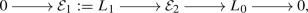(21)

where $${{\mathcal {E}}}_2$$ is a rank-two vector bundle, necessarily Ulrich, as $$L_0$$ and $$L_1$$ are. We proceed taking extensions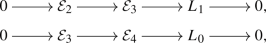and so on; that is, defining

\begin{aligned} \epsilon _r= {\left\{ \begin{array}{ll} 0, &{} \hbox {if}\, \, r\, \, \hbox {is even}, \\ 1, &{} \hbox {if}\,\, r \,\, \hbox {is odd}, \end{array}\right. } \end{aligned}
(22)

we take successive extensions $$[{{\mathcal {E}}}_{r+1}] \in {\text {Ext}}^1(L_{\epsilon _{r+1}},{{\mathcal {E}}}_r)$$ for all $$r \geqslant 1$$: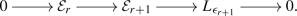(23)

A priori we do not know that we can always take non-split such extensions; this we will prove in a moment. In any case, all $${{\mathcal {E}}}_{r}$$ are Ulrich vector bundles of rank r, as extensions of Ulrich bundles are again Ulrich.

### Lemma 5.2

Let L be $$L_0$$ or $$L_1$$. Then, for all $$r \geqslant 1$$ we have

1. (i)

$$h^2({{\mathcal {E}}}_r \otimes L^*)=0$$,

2. (ii)

$$h^2({{\mathcal {E}}}_r^* \otimes L)=0$$,

3. (iii)

$$h^1({{\mathcal {E}}}_r \otimes L_{\epsilon _{r+1}}^*)\geqslant h \geqslant 3$$.

### Proof

We prove (i)–(ii) by induction on r. Assertion (i) follows from Lemma 5.1 because $${{\mathcal {E}}}_1=L_1$$. Assuming it holds for r, we have, by tensoring (23) by $$L^*$$, that

\begin{aligned} h^2({{\mathcal {E}}}_{r+1} \otimes L^*) \leqslant h^2({{\mathcal {E}}}_r \otimes L^*)+h^2(L_{\epsilon _{r+1}} \otimes L^*)=0, \end{aligned}

by the induction hypothesis and Lemma 5.1, since $$L_{\epsilon _{r+1}} \otimes L^*$$ equals one of $${{\mathcal {O}}}_X$$, $$L_0-L_1$$ or $$L_1-L_0$$.

A similar reasoning, tensoring the dual of (23) by L, proves (ii).

To prove (iii), first note that it holds for $$r=1$$, as $$h^1({{\mathcal {E}}}_1 \otimes L_{\epsilon _{2}}^*)=h^1(L_1-L_0)= h$$ by Lemma 5.1. Then, for any $$r \geqslant 1$$, tensor (23) by $$L_{\epsilon _{r+2}}^*$$. Using that $$h^2({{\mathcal {E}}}_r\otimes L_{\epsilon _{r+2}}^*)=0$$ by (i), we see that $$h^1({{\mathcal {E}}}_{r+1} \otimes L_{\epsilon _{r+2}}^*)\geqslant h^1(L_{\epsilon _{r+1}}\otimes L_{\epsilon _{r+2}}^*)=h \geqslant 3$$, by Lemma 5.1. $$\square$$

By (iii) of the last lemma, we have that $$\dim ({\text {Ext}}^1(L_{\epsilon _{r+1}},{{\mathcal {E}}}_r))=h^1({{\mathcal {E}}}_r \otimes L_{\epsilon _{r+1}}^*)>0$$ for all $$r \geqslant 1$$, which means that we can always pick non-split extensions of the form (23). We will henceforth do so.

### Lemma 5.3

For all $$r \geqslant 1$$ we have

1. (i)

$$h^1({{\mathcal {E}}}_{r+1} \otimes L_{\epsilon _{r+1}}^*)=h^1({{\mathcal {E}}}_r \otimes L_{\epsilon _{r+1}}^*)-1$$,

2. (ii)

$$h^1({{\mathcal {E}}}_r \otimes L_{\epsilon _{r+1}}^*)=\lfloor \frac{r+1}{2}\rfloor (h-1) +1$$,

3. (iii)

$$h^2({{\mathcal {E}}}_r \otimes {{\mathcal {E}}}_r^*)=0$$,

4. (iv)

$$\chi ({{\mathcal {E}}}_r \otimes L_{\epsilon _{r+1}}^*)=-\left\lfloor \frac{r+1}{2}\right\rfloor (h-1)-\epsilon _r$$,

5. (v)

$$\chi (L_{\epsilon _{r}} \otimes {{\mathcal {E}}}_r^* )=-\left\lfloor \frac{r+1}{2}\right\rfloor (h-1)+\epsilon _rh$$,

6. (vi)

$$\chi ({{\mathcal {E}}}_r \otimes {{\mathcal {E}}}_r^*)=-\frac{1}{2}\left( r^2-\epsilon _r\right) \left( h-1\right) +\epsilon _r$$.

7. (vii)

the slope of $${{\mathcal {E}}}_r$$ is $$\mu ({{\mathcal {E}}}_r)=L_0\cdot \xi _{m,n}=L_1\cdot \xi _{m,n}$$.

### Proof

(i) Since $${\text {Ext}}^1(L_{\epsilon _{r+1}},{{\mathcal {E}}}_r)\simeq H^1({{\mathcal {E}}}_r \otimes L_{\epsilon _{r+1}}^*)$$ and the sequence (23) is constructed by taking a nonzero element therein, the coboundary map $$H^0({{\mathcal {O}}}_X) \rightarrow H^1({{\mathcal {E}}}_r \otimes L_{\epsilon _{r+1}}^*)$$ of (23) tensored by $$L_{\epsilon _{r+1}}^*$$, i.e.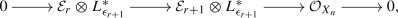(24)

is nonzero. Thus, (i) follows from the cohomology of (24).

(ii) We use induction on r. For $$r=1$$, the right-hand side of the formula yields h, whereas the left-hand side equals $$h^1({{\mathcal {E}}}_1 \otimes L_0^*)=h^1(L_1-L_0)$$; thus the formula is correct by Lemma 5.1.

Assume now that the formula holds for r. Tensoring (23) by $$L_{\epsilon _{r+2}}^*$$, we obtain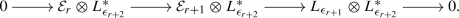(25)

We have $$h^0(L_{\epsilon _{r+1}}\otimes L_{\epsilon _{r+2}}^*)=0$$ and $$h^1(L_{\epsilon _{r+1}}\otimes L_{\epsilon _{r+2}}^*)=h$$ by Lemma 5.1, and $$h^2({{\mathcal {E}}}_r\otimes L_{\epsilon _{r+2}}^*)=0$$ by Lemma 5.2. Thus,

\begin{aligned} h^1({{\mathcal {E}}}_{r+1} \otimes L_{\epsilon _{r+2}}^*)=h+h^1({{\mathcal {E}}}_r\otimes L_{\epsilon _{r+2}}^*)=h+h^1({{\mathcal {E}}}_r\otimes L_{\epsilon _{r}}^*) \end{aligned}

Using (i) and the induction hypothesis, this equals

\begin{aligned} h+\left( h^1({{\mathcal {E}}}_{r-1} \otimes L_{\epsilon _{r}}^*)-1\right)&= h+\left( \left\lfloor \frac{(r-1)+1}{2}\right\rfloor (h-1) +1\right) -1\\&= \left\lfloor \frac{(r+1)+1}{2}\right\rfloor (h-1)+1, \end{aligned}

showing that the formula holds for $$r+1$$.

(iii) We again use induction on r. For $$r=1$$ (iii) says that $$h^2(L_1 -L_1)=h^2({{\mathcal {O}}}_{X_n})=0$$, which is true. Assume now that (iii) holds for r. The cohomology of (23) tensored by $${{\mathcal {E}}}_{r+1}^*$$ and Lemma 5.2(ii) yield

\begin{aligned} h^2( {{\mathcal {E}}}_{r+1} \otimes {{\mathcal {E}}}_{r+1}^*) \leqslant h^2( {{\mathcal {E}}}_{r} \otimes {{\mathcal {E}}}_{r+1}^*) + h^2( L_{\epsilon _{r+1}} \otimes {{\mathcal {E}}}_{r+1}^*) =h^2( {{\mathcal {E}}}_{r} \otimes {{\mathcal {E}}}_{r+1}^*). \end{aligned}
(26)

The cohomology of the dual of (23) tensored by $${{\mathcal {E}}}_{r}$$ and Lemma 5.2(i) yields

\begin{aligned} h^2( {{\mathcal {E}}}_{r} \otimes {{\mathcal {E}}}_{r+1}^*) \leqslant h^2({{\mathcal {E}}}_r \otimes L_{\epsilon _{r+1}}^*)+h^2({{\mathcal {E}}}_{r} \otimes {{\mathcal {E}}}_{r}^*)= h^2({{\mathcal {E}}}_{r} \otimes {{\mathcal {E}}}_{r}^*). \end{aligned}
(27)

Now (26)–(27) and the induction hypothesis yield $$h^2( {{\mathcal {E}}}_{r+1} \otimes {{\mathcal {E}}}_{r+1}^*)=0$$, as desired.

(iv) For $$r=1$$ (iv) reads $$\chi (L_1 -L_0)=-h$$, which is true by Lemma 5.1. For $$r=2$$ (iv) reads $$\chi ({{\mathcal {E}}}_2 \otimes L_1^*)=-h+1$$; using sequence (25) with $$r=1$$, one computes $$\chi ({{\mathcal {E}}}_2 \otimes L_1^*)=\chi ({{\mathcal {O}}}_{X_n})+\chi (L_0-L_1)=1-h$$, by Lemma 5.1, so again the formula is correct.

Assume now that the formula holds up to a certain $$r\geqslant 2$$. From (25) and Lemma 5.1 we find

\begin{aligned} \chi ({{\mathcal {E}}}_{r+1}\otimes L_{\epsilon _{r+2}}^*) = \chi ({{\mathcal {E}}}_r\otimes L_{\epsilon _{r+2}}^*)+ \chi (L_{\epsilon _{r+1}}\otimes L_{\epsilon _{r+2}}^*)= \chi ({{\mathcal {E}}}_r\otimes L_{\epsilon _{r}}^*)-h. \end{aligned}
(28)

Then (24) (with r replaced by $$r-1$$) yields

\begin{aligned} \chi ({{\mathcal {E}}}_r\otimes L_{\epsilon _{r}}^*)=\chi ({{\mathcal {E}}}_{r-1}\otimes L_{\epsilon _{r}}^*)+\chi ({{\mathcal {O}}}_{X_n})= \chi ({{\mathcal {E}}}_{r-1}\otimes L_{\epsilon _{r}}^*)+1. \end{aligned}
(29)

Inserting into (28) and using the induction hypothesis, we get

\begin{aligned} \chi ({{\mathcal {E}}}_{r+1}\otimes L_{\epsilon _{r+2}}^*)= & {} \chi ({{\mathcal {E}}}_{r-1}\otimes L_{\epsilon _{r}}^*)+1-h \\= & {} -(h-1)\left\lfloor \frac{(r-1)+1}{2}\right\rfloor -\epsilon _{r-1}+1-h \\= & {} -(h-1)\left( \left\lfloor \frac{r}{2}\right\rfloor +1\right) -\epsilon _{r-1}\\= & {} -(h-1)\left\lfloor \frac{(r+1)+1}{2}\right\rfloor -\epsilon _{r+1}, \end{aligned}

proving that the formula holds also for $$r+1$$.

(v) For $$r=1$$ (v) reads $$\chi (L_1 -L_1)=1$$, which is true. For $$r=2$$ (v) reads $$\chi (L_0\otimes {{\mathcal {E}}}_2^*)=-h+1$$; from the dual of sequence (21) tensored by $$L_0$$, one computes $$\chi (L_0\otimes {{\mathcal {E}}}_2^*)=\chi ({{\mathcal {O}}}_{X_n})+\chi (L_0-L_1)=1-h$$, by Lemma 5.1, so again the formula is correct.

Assume now that the formula holds up to a certain $$r\geqslant 2$$. From the dual of sequence (23) tensored by $$L_{\epsilon _{r+1}}$$ we find

\begin{aligned} \chi (L_{\epsilon _{r+1}} \otimes {{\mathcal {E}}}_{r+1}^* )= & {} \chi (L_{\epsilon _{r+1}} \otimes L_{\epsilon _{r+1}}^*)+ \chi (L_{\epsilon _{r+1}}\otimes {{\mathcal {E}}}_{r}^*) \nonumber \\= & {} \chi ({{\mathcal {O}}}_{X_n})+\chi (L_{\epsilon _{r+1}}\otimes {{\mathcal {E}}}_{r}^*) = 1+\chi (L_{\epsilon _{r+1}}\otimes {{\mathcal {E}}}_{r}^*). \end{aligned}
(30)

The dual of sequence (23) with r replaced by $$r-1$$ tensored by $$L_{\epsilon _{r+1}}$$, together with Lemma 5.1, yields

\begin{aligned} \chi (L_{\epsilon _{r+1}}\otimes {{\mathcal {E}}}_{r}^*)=\chi (L_{\epsilon _{r+1}} \otimes L_{\epsilon _{r}}^*)+ \chi (L_{\epsilon _{r+1}}\otimes {{\mathcal {E}}}_{r-1}^*)= -h+\chi (L_{\epsilon _{r-1}}\otimes {{\mathcal {E}}}_{r-1}^*). \end{aligned}
(31)

Inserting into (30) and using the induction hypothesis, we get

\begin{aligned} \chi (L_{\epsilon _{r+1}} \otimes {{\mathcal {E}}}_{r+1}^* )= & {} 1-h+\chi (L_{\epsilon _{r-1}}\otimes {{\mathcal {E}}}_{r-1}^*) \\= & {} 1-h-(h-1)\left\lfloor \frac{(r-1)+1}{2}\right\rfloor +\epsilon _{r-1}h \\= & {} -(h-1)\left( \left\lfloor \frac{r}{2}\right\rfloor +1\right) +\epsilon _{r-1}h\\= & {} -(h-1)\left\lfloor \frac{(r+1)+1}{2}\right\rfloor +\epsilon _{r+1}h, \end{aligned}

proving that the formula holds also for $$r+1$$.

(vi) We check the given formula for $$r=1,2$$.

We have $$\chi ({{\mathcal {E}}}_1 \otimes {{\mathcal {E}}}_1^*)=\chi (L_1-L_1)=\chi ({{\mathcal {O}}}_X)=1$$, which fits with the given formula for $$r=1$$.

From (21) tensored by $${{\mathcal {E}}}_2^*$$ we get

\begin{aligned} \chi ({{\mathcal {E}}}_2 \otimes {{\mathcal {E}}}_2^*)=\chi (L_1 \otimes {{\mathcal {E}}}_2^*)+\chi (L_0 \otimes {{\mathcal {E}}}_2^*){\mathop {=}\limits ^{(v)}} \chi (L_1 \otimes {{\mathcal {E}}}_2^*)-(h-1). \end{aligned}
(32)

From the dual of (21) tensored by $$L_1$$ and Lemma 5.1 we get

\begin{aligned} \chi (L_1 \otimes {{\mathcal {E}}}_2^*)=\chi (L_1 -L_1)+\chi (L_1 -L_0)=\chi ({{\mathcal {O}}}_{X_n})-h=1-h. \end{aligned}
(33)

Combining (32) and (33), we get $$\chi ({{\mathcal {E}}}_2 \otimes {{\mathcal {E}}}_2^*)=-2(h-1)$$, which again fits with the given formula for $$r=2$$.

Assume now that the given formula is valid up to a certain $$r\geqslant 2$$. From (23) tensored by $${{\mathcal {E}}}_{r+1}^*$$ and successively the dual of (23) tensored by $${{\mathcal {E}}}_r$$ we get

\begin{aligned} \chi ({{\mathcal {E}}}_{r+1} \otimes {{\mathcal {E}}}_{r+1}^*)= & {} \chi ({{\mathcal {E}}}_{r} \otimes {{\mathcal {E}}}_{r+1}^*)+ \chi (L_{\epsilon _{r+1}} \otimes {{\mathcal {E}}}_{r+1}^*) \\= & {} \chi ({{\mathcal {E}}}_{r} \otimes {{\mathcal {E}}}_{r}^*) +\chi ({{\mathcal {E}}}_{r} \otimes L_{\epsilon _{r+1}}^*)+ \chi (L_{\epsilon _{r+1}} \otimes {{\mathcal {E}}}_{r+1}^*). \end{aligned}

Using the induction hypothesis, together with (iv) and (v) (with r substituted by $$r+1$$), the right-hand side can be written as

\begin{aligned} \left[ -\frac{1}{2}\left( r^2-\epsilon _r\right) \left( h-1\right) +\epsilon _r\right] +\left[ -(h-1)\left\lfloor \frac{r+1}{2}\right\rfloor -\epsilon _r\right] +\left[ -(h-1)\left\lfloor \frac{r+2}{2}\right\rfloor +\epsilon _{r+1}h\right] \end{aligned}

An easy computation shows that this equals

\begin{aligned} -\frac{1}{2}\left( (r+1)^2-\epsilon _{r+1}\right) \left( h-1\right) +\epsilon _{r+1}, \end{aligned}

finishing the inductive step.

(vii) This can either easily be checked by induction again or follows from the fact that the slope of an Ulrich bundle only depends on the ambient polarized variety, cf. e.g. [6, Prop. 2.1(3)], and must therefore equal the slope of $$L_0$$ and $$L_1$$ for all ranks. $$\square$$

We now define, for each $$r \geqslant 1$$, the scheme $$\mathfrak {U}(r)$$ to be the modular family of the vector bundles $${{\mathcal {E}}}_r$$ defined above. For $$r \geqslant 2$$, the scheme $$\mathfrak {U}(r)$$ contains a subscheme $$\mathfrak {U}(r)^\textrm{ext}$$ parametrizing bundles $${{\mathcal {F}}}_r$$ that are non-split extensions of the form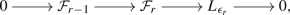(34)

with $$[{{\mathcal {F}}}_{r-1}] \in \mathfrak {U}(r-1)$$.

### Lemma 5.4

Let $${{\mathcal {F}}}_r$$ be a general member of $$\mathfrak {U}(r)$$. Then $${{\mathcal {F}}}_r$$ is Ulrich of rank r with slope $$\mu :=L_0\cdot \xi _{m,n}=L_1\cdot \xi _{m,n}$$.

Moreover,

1. (i)

$$\chi ({{\mathcal {F}}}_r \otimes {{\mathcal {F}}}_r^*)=-\frac{1}{2}\left( r^2-\epsilon _r\right) \left( h-1\right) +\epsilon _r$$,

2. (ii)

$$h^2({{\mathcal {F}}}_r \otimes {{\mathcal {F}}}_r^*)=0$$,

3. (iii)

$$h^1({{\mathcal {F}}}_r \otimes L_{\epsilon _{r+1}}^*) \leqslant \lfloor \frac{r+1}{2}\rfloor (h-1) +1$$.

### Proof

Ulrichness is an open property in the family, and the rank and slope are constant, so the general member of $$\mathfrak {U}(r)$$ is Ulrich of rank r and slope $$\mu$$ as each $${{\mathcal {E}}}_r$$ constructed above is (cf. Lemma 4.3(vii)) or use the fact that the slope of an Ulrich bundle only depends on the ambient polarized variety, cf. e.g. [6, Prop. 2.1(3)]).

Properties (ii) and (iii) follows by specializing $${{\mathcal {F}}}_r$$ to an $${{\mathcal {E}}}_r$$ constructed above, and using semicontinuity and Lemma 5.3(iii) and (ii), respectively. Property (i) follows by Lemma 5.3(vi), since the given $$\chi$$ depends only on the Chern classes of the two factors and of $$X_n$$, which are constant in the family $$\mathfrak {U}(r)$$. $$\square$$

We wish to prove that the general member of $$\mathfrak {U}(r)$$ is slope-stable. To this end we will need a couple of auxiliary results.

### Lemma 5.5

Let $$r \geqslant 2$$ and assume that $$[{{\mathcal {F}}}_r] \in \mathfrak {U}(r)^\textrm{ext}$$ sits in a non-split sequence like (34) with $$[{{\mathcal {F}}}_{r-1}] \in \mathfrak {U}(r-1)$$ being slope-stable. Then

1. (i)

$${{\mathcal {F}}}_r$$ is simple (that is, $$h^0({{\mathcal {F}}}_r \otimes {{\mathcal {F}}}_r^*)=1$$);

2. (ii)

if $${{\mathcal {G}}}$$ is a destabilizing subsheaf of $${{\mathcal {F}}}_r$$, then $${{\mathcal {G}}}^{*} \simeq {{\mathcal {F}}}_{r-1}^*$$ and $$({{\mathcal {F}}}_r/{{\mathcal {G}}})^* \simeq L_{\epsilon _r}^*$$; if, furthermore, $${{\mathcal {F}}}_r/{{\mathcal {G}}}$$ is torsion-free, then $${{\mathcal {G}}}\simeq {{\mathcal {F}}}_{r-1}$$ and $${{\mathcal {F}}}_r/{{\mathcal {G}}}\simeq L_{\epsilon _r}$$.

### Proof

We first prove (ii).

Assume that $${{\mathcal {G}}}$$ is a destabilizing subsheaf of $${{\mathcal {F}}}_r$$, that is $$0<{\text {rk}}({{\mathcal {G}}}) < {\text {rk}}({{\mathcal {F}}}_r)=r$$ and $$\mu ({{\mathcal {G}}}) \geqslant \mu :=\mu ({{\mathcal {F}}}_r)$$. Define

\begin{aligned} {{\mathcal {Q}}}:={\text {im}}\{{{\mathcal {G}}}\subset {{\mathcal {F}}}_r \rightarrow L_{\epsilon _r}\} \; \; \text{ and } \; \; {{\mathcal {K}}}:=\ker \{{{\mathcal {G}}}\rightarrow {{\mathcal {Q}}}\}. \end{aligned}

Then we may put (34) into a commutative diagram with exact rows and columns: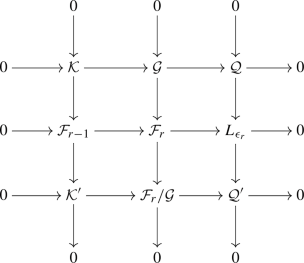defining $${{\mathcal {K}}}'$$ and $${{\mathcal {Q}}}'$$. We have $${\text {rk}}({{\mathcal {Q}}}) \leqslant 1$$.

Assume that $${\text {rk}}({{\mathcal {Q}}})=0$$. Then $${{\mathcal {Q}}}=0$$, whence $${{\mathcal {K}}}\simeq {{\mathcal {G}}}$$ and $${{\mathcal {Q}}}' \simeq L_{\epsilon _r}$$. Since $$\mu ({{\mathcal {K}}}) =\mu ({{\mathcal {G}}}) \geqslant \mu =\mu ({{\mathcal {F}}}_{r-1})$$ and $${{\mathcal {F}}}_{r-1}$$ is slope-stable, we must have $${\text {rk}}({{\mathcal {K}}})={\text {rk}}({{\mathcal {F}}}_{r-1})=r-1$$. It follows that $${\text {rk}}({{\mathcal {K}}}')=0$$. Since

\begin{aligned} c_1({{\mathcal {K}}}) = c_1({{\mathcal {F}}}_{r-1})-c_1({{\mathcal {K}}}')=c_1({{\mathcal {F}}}_{r-1})-D', \end{aligned}

where $$D'$$ is an effective divisor supported on the codimension one locus of the support of $${{\mathcal {K}}}'$$, we have

\begin{aligned} \mu \leqslant \mu ({{\mathcal {K}}})=\frac{\left( c_1({{\mathcal {F}}}_{r-1})-D'\right) \cdot \xi _{m,n}}{r-1}= \frac{c_1({{\mathcal {F}}}_{r-1})\cdot \xi _{m,n}}{r-1}-\frac{D' \cdot \xi _{m,n}}{r-1} = \mu -\frac{D' \cdot \xi _{m,n}}{r-1}. \end{aligned}

Hence $$D'=0$$, which means that $${{\mathcal {K}}}'$$ is supported in codimension at least two. Thus, $$\mathcal {E}xt^i({{\mathcal {K}}}',{{\mathcal {O}}}_X)=0$$ for $$i \leqslant 1$$, and it follows that $${{\mathcal {G}}}^* \simeq {{\mathcal {K}}}^* \simeq {{\mathcal {F}}}_{r-1}^*$$ and $$({{\mathcal {F}}}_r/{{\mathcal {G}}})^* \simeq {{{\mathcal {Q}}}'}^* \simeq L_{\epsilon _r}^*$$, as desired. If, furthermore, $${{\mathcal {F}}}_r/{{\mathcal {G}}}$$ is torsion-free, then we must have $${{\mathcal {K}}}'=0$$, whence $${{\mathcal {G}}}\simeq {{\mathcal {F}}}_{r-1}$$ and $${{\mathcal {F}}}_r/{{\mathcal {G}}}\simeq L_{\epsilon _r}$$.

Next we prove that $${\text {rk}}({{\mathcal {Q}}})=1$$ cannot happen. Indeed, if $${\text {rk}}({{\mathcal {Q}}})=1$$, then $${\text {rk}}({{\mathcal {K}}})={\text {rk}}({{\mathcal {G}}})-1 \leqslant r-2<r-1={\text {rk}}({{\mathcal {F}}}_{r-1})$$ and $${\text {rk}}({{\mathcal {Q}}}')=0$$; in particular, $${{\mathcal {Q}}}'$$ is a torsion sheaf. Since

\begin{aligned} c_1({{\mathcal {K}}}) = c_1({{\mathcal {G}}})-c_1({{\mathcal {Q}}})=c_1({{\mathcal {G}}})-c_1(L_{\epsilon _r})+c_1({{\mathcal {Q}}}')=c_1({{\mathcal {G}}})-c_1(L_{\epsilon _r})+D, \end{aligned}

where D is an effective divisor supported on the codimension one locus of the support of $${{\mathcal {Q}}}'$$, we have

\begin{aligned} \mu ({{\mathcal {K}}})= & {} \frac{\Big (c_1({{\mathcal {G}}})-c_1(L_{\epsilon _r})+D\Big ) \cdot \xi _{m,n}}{{\text {rk}}({{\mathcal {K}}})} \geqslant \frac{\Big (c_1({{\mathcal {G}}})-c_1(L_{\epsilon _r})\Big ) \cdot \xi _{m,n}}{{\text {rk}}({{\mathcal {K}}})}\\= & {} \frac{\mu ({{\mathcal {G}}}){\text {rk}}({{\mathcal {G}}})-c_1(L_{\epsilon _r})\cdot \xi _{m,n}}{{\text {rk}}({{\mathcal {K}}})} = \frac{\mu ({{\mathcal {G}}}){\text {rk}}({{\mathcal {G}}})-\mu }{{\text {rk}}({{\mathcal {G}}})-1} \geqslant \frac{\mu {\text {rk}}({{\mathcal {G}}})-\mu }{{\text {rk}}({{\mathcal {G}}})-1} =\mu \end{aligned}

This contradicts slope-stability of $${{\mathcal {F}}}_{r-1}$$.

To prove (i), assume that $${{\mathcal {F}}}_r$$ is non-simple, that is, it admits a nontrivial endomorphism. Then there exists a nonzero endomorphism $$\varphi :{{\mathcal {F}}}_r \rightarrow {{\mathcal {F}}}_r$$ dropping rank everywhere. Indeed, take any endomorphism $$\alpha$$ that is not a constant times the identity, pick an eigenvalue $$\lambda$$ of $$\alpha (x)$$ for some $$x \in X_n$$ and set $$\varphi =\alpha -\lambda {\text {id}}$$; then $${\text {det}}(\varphi ) \in H^0({\text {det}}({{\mathcal {F}}}_r^*) \otimes {\text {det}}({{\mathcal {F}}}_r))=H^0({{\mathcal {O}}}_{X_n})\simeq {{\mathbb {C}}}$$ vanishes at x, whence it is identically zero. Both $$\ker (\varphi )$$ and $${\text {im}}(\varphi )$$, being subsheaves of $${{\mathcal {F}}}_r$$, are torsion-free, and the equality

\begin{aligned} {\text {rk}}({\mathcal {F}}_{r})\mu ({\mathcal {F}}_{r})={\text {rk}}( \textrm{ker}(\varphi ))\mu (\textrm{ker}(\varphi ))+{\text {rk}}(\textrm{im}(\varphi ))\mu ( \textrm{im}(\varphi )) \end{aligned}

easily yields that one of them is destabilizing. By part (ii), it follows that either $$\ker (\varphi )\simeq {{\mathcal {F}}}_{r-1}$$ (and $${\text {im}}(\varphi )\simeq L_{\epsilon _r})$$ or $${\text {im}}(\varphi )^* \simeq {{\mathcal {F}}}_{r-1}^*$$. In the first case, if $$\varphi ^2=0$$, then $$L_{\epsilon _r} \subset {{\mathcal {F}}}_{r+1}$$, contradicting the slope-stability of $${{\mathcal {F}}}_{r+1}$$, and if $$\varphi ^2 \ne 0$$, then $$\varphi$$ maps $$L_{\epsilon _r}$$ isomorphically to $$L_{\epsilon _r}$$, implying that (34) splits, a contradiction. In the second case, if $$\varphi ^2=0$$, then $$r=2$$, $${{\mathcal {F}}}_{r-1} =L_1 ={\text {im}}(\varphi ) \subset \ker (\varphi ) =L_0$$, yielding the contradiction $$h^0(L_0-L_1)>0$$, whereas if $$\varphi ^2 \ne 0$$, then, since $${{\mathcal {F}}}_{r+1}$$ is slope-stable, $$\varphi$$ maps $${{\mathcal {F}}}_{r+1}$$ isomorphically to $${{\mathcal {F}}}_{r+1}$$, implying again that (34) splits, a contradiction. $$\square$$

### Lemma 5.6

Let $$r \geqslant 2$$ and assume that the general member of $$\mathfrak {U}(r-1)$$ is slope-stable. Then $$\mathfrak {U}(r)$$ is generically smooth of dimension $$\frac{1}{2}(r^2-\epsilon _r)(h-1)+\epsilon _{r+1}$$ and properly contains $$\mathfrak {U}(r)^\textrm{ext}$$.

### Proof

The general member $${{\mathcal {F}}}_r$$ of $$\mathfrak {U}(r)$$ satisfies $$h^0({{\mathcal {F}}}_r \otimes {{\mathcal {F}}}_r^*)=1$$ by Lemma 5.5(i) and $$h^2({{\mathcal {F}}}_r \otimes {{\mathcal {F}}}_r^*)=0$$ by Lemma 5.4(ii). Hence, one has (see e.g. [5, Prop. 2.10]) that $$\mathfrak {U}(r)$$ is generically smooth of dimension

\begin{aligned} h^1({{\mathcal {F}}}_r \otimes {{\mathcal {F}}}_r^*)= & {} -\chi ({{\mathcal {F}}}_r \otimes {{\mathcal {F}}}_r^*)+h^0({{\mathcal {F}}}_r \otimes {{\mathcal {F}}}_r^*)+h^2({{\mathcal {F}}}_r \otimes {{\mathcal {F}}}_r^*)=-\chi ({{\mathcal {F}}}_r \otimes {{\mathcal {F}}}_r^*)+1 \\= & {} \frac{1}{2}\left( r^2-\epsilon _r\right) \left( h-1\right) -\epsilon _r+1 =\frac{1}{2}\left( r^2-\epsilon _r\right) \left( h-1\right) +\epsilon _{r+1}, \end{aligned}

where we have used Lemma 5.4(i), as claimed.

Similarly, being slope-stable, also the general member $${{\mathcal {F}}}_{r-1}$$ of $$\mathfrak {U}(r-1)$$ satisfies $$h^0({{\mathcal {F}}}_{r-1} \otimes {{\mathcal {F}}}_{r-1}^*)=1$$, so the same reasoning shows that

\begin{aligned} \dim (\mathfrak {U}(r-1))= \frac{1}{2}\left( (r-1)^2-\epsilon _{r-1}\right) \left( h-1\right) +\epsilon _{r}. \end{aligned}
(35)

Moreover,

\begin{aligned} \dim ({\text {Ext}}^1(L_{\epsilon _r},{{\mathcal {F}}}_{r-1}))=h^1({{\mathcal {F}}}_{r-1} \otimes L_{\epsilon _r}^*)\leqslant \left\lfloor \frac{r}{2}\right\rfloor (h-1) +1. \end{aligned}
(36)

by Lemma 5.4(iii). Hence,

\begin{aligned} \dim (\mathfrak {U}(r)^\textrm{ext})\leqslant & {} \dim (\mathfrak {U}(r-1))+\dim {{\mathbb {P}}}({\text {Ext}}^1(L_{\epsilon _r},{{\mathcal {F}}}_{r-1})) \\\leqslant & {} \frac{1}{2}\left( (r-1)^2-\epsilon _{r-1}\right) \left( h-1\right) +\epsilon _{r} +\left\lfloor \frac{r}{2}\right\rfloor (h-1) \\= & {} \frac{1}{2} \left( r^2-\epsilon _{r}\right) \left( h-1\right) +\epsilon _{r+1}-\left( \left\lfloor \frac{r+1}{2} \right\rfloor -\epsilon _r\right) (h-1)+\epsilon _r-\epsilon _{r+1} \\= & {} \dim (\mathfrak {U}(r)) - \left( \left\lfloor \frac{r+1}{2} \right\rfloor -\epsilon _r\right) (h-1)+\epsilon _r-\epsilon _{r+1}, \end{aligned}

and one easily sees that this is strictly less than $$\dim (\mathfrak {U}(r))$$, since $$r \geqslant 2$$ and $$h \geqslant 3$$. Thus, $$\mathfrak {U}(r)^\textrm{ext}$$ is properly contained in $$\mathfrak {U}(r)$$, as claimed. $$\square$$

We can now prove slope-stability of the general member of $$\mathfrak {U}(r)$$.

### Proposition 5.7

Let $$r\geqslant 1$$. The general member of $$\mathfrak {U}(r)$$ is slope-stable.

### Proof

We use induction on r, the result being trivially true for $$r=1$$. Assume $$r \geqslant 2$$ and that the general member of $$\mathfrak {U}(r)$$ is not slope-stable, whereas the general member of $$\mathfrak {U}(r-1)$$ is. Consider a one-parameter flat family $${{\mathcal {F}}}\rightarrow {{\mathbb {D}}}$$ of bundles over the disc $${{\mathbb {D}}}$$ such that the generic member $${{\mathcal {F}}}^{(t)}$$ of this family is also a generic member of $$\mathfrak {U}(r)$$ and the fiber $${{\mathcal {F}}}^{(0)}$$ of $${{\mathcal {F}}}\rightarrow {{\mathbb {D}}}$$ over $$0\in {{\mathbb {D}}}$$ lies in $$\mathfrak {U}(r)^\textrm{ext}$$. Since we are assuming that that the generic member of $$\mathfrak {U}(r)$$ is not slope-stable, we have that $${{\mathcal {F}}}^{(t)}$$ is not stable so that there is a destabilizing sequence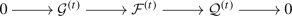(37)

which we can take to be saturated, that is, such that $${{\mathcal {Q}}}^{(t)}$$ is torsion free, whence so that $${{\mathcal {G}}}^{(t)}$$ and $${{\mathcal {Q}}}^{(t)}$$ are (Ulrich) vector bundles (see [5, Thm. 2.9] or [4, (3.2)]). We may also assume that, up to base change, the sequence (37) is defined over the field of meromorphic functions over $${{\mathbb {D}}}$$, so that there are two flat families $${{\mathcal {G}}}\rightarrow {{\mathbb {D}}}$$ and $${{\mathcal {Q}}}\rightarrow {{\mathbb {D}}}$$ of bundles over the disc $${{\mathbb {D}}}$$ such that the generic members of $${{\mathcal {G}}}\rightarrow {{\mathbb {D}}}$$ and $${{\mathcal {Q}}}\rightarrow {{\mathbb {D}}}$$ are $${{\mathcal {G}}}^{(t)}$$ and $${{\mathcal {Q}}}^{(t)}$$, respectively.

The limit of $${{\mathbb {P}}}({{\mathcal {Q}}}^{(t)}) \subset {{\mathbb {P}}}({{\mathcal {F}}}^{(t)})$$ defines a subvariety of $${{\mathbb {P}}}({{\mathcal {F}}}^{(0)})$$ of the same dimension as $${{\mathbb {P}}}({{\mathcal {Q}}}^{(t)})$$, whence a coherent sheaf $${{\mathcal {Q}}}^{(0)}$$ of rank $${\text {rk}}({{\mathcal {Q}}}^{(t)})$$ with a surjection $${{\mathcal {F}}}^{(0)} \rightarrow {{\mathcal {Q}}}^{(0)}$$. Denoting by $${{\mathcal {G}}}^{(0)}$$ its kernel, we have $${\text {rk}}({{\mathcal {G}}}^{(0)})={\text {rk}}({{\mathcal {G}}}^{(t)})$$ and $$c_1({{\mathcal {G}}}^{(0)})=c_1({{\mathcal {G}}}^{(t)})$$. Hence, (37) specializes to a destabilizing sequence for $$t=0$$. Lemma 5.5 yields that $${{{\mathcal {G}}}^{(0)}}^*$$ (respectively, $${{{\mathcal {Q}}}^{(0)}}^*$$) is the dual of a member of $$\mathfrak {U}(r-1)$$ (resp., the dual of $$L_{\epsilon _r}$$). It follows that $${{{\mathcal {G}}}^{(t)}}^*$$ (resp., $${{{\mathcal {Q}}}^{(t)}}^*$$) is a deformation of the dual of a member of $$\mathfrak {U}(r-1)$$ (resp., a deformation of $$L_{\epsilon _r}^*$$), whence that $${{\mathcal {G}}}^{(t)}$$ is a deformation of a member of $$\mathfrak {U}(r-1)$$, as both are locally free, and $${{\mathcal {Q}}}^{(t)} \simeq L_{\epsilon _r}$$, for the same reason.

In other words, the general member of $$\mathfrak {U}(r)$$ is an extension of $$L_{\epsilon _r}$$ by a member of $$\mathfrak {U}(r-1)$$. Hence, $$\mathfrak {U}(r)=\mathfrak {U}(r)^\textrm{ext}$$, contradicting Lemma 5.6. $$\square$$

By combining Proposition 5.7 and Lemma 5.4 we deduce:

### Theorem 5.8

For any $$r \geqslant 1$$, the blown-up plane $$X_n$$, with $$n\geqslant 2$$, carries slope-stable rank-r Ulrich bundles, and their moduli space contains a reduced and irreducible component of dimension $$\frac{1}{2}(r^2-\epsilon _r)(h-1)+\epsilon _{r+1}$$.